# Bihar Board 12th Physics Objective Answers Chapter 9 Ray Optics and Optical Instruments

Bihar Board 12th Physics Objective Questions and Answers

## Bihar Board 12th Physics Objective Answers Chapter 9 Ray Optics and Optical Instruments

Question 1.
What can be the largest distance of an image of a real object from a convex mirror of radius of curvature is 20 cm ?
(a) 10 cm
(b) 20cm
(c) Infinity
(d) Zero
(a) 10 cmQuestion 2.
A boy of height 1 m stands in front of a convex mirror. His distance from the mirror is equal to its focal length. The height of his image is
(a) 0.25 m
(b) 0.33 m
(c) 0.5m
(d) 0.67 m
(c) 0.5m
Solution:
(c) Let/be focal length of the convex mirror. According to new cartesian sign convention Object distance, u = -f focal length = +fQuestion 3.
An object 2 cm high is placed at a distance of 16 cm from a concave mirror, which produces a real image 3 cm high. What is the focal length of the mirror ?
(a) -9.6 cm
(b) -3.6 cm
(c) -6.3 cm
(d) -8.3 cm
(a) -9.6 cmQuestion 4.
(a) Travel as a cylindrical beam
(b) Diverge
(c) Converge
(d) Diverge near the axis and converge near the periphery
(c) Converge

Question 5.
A ray of light strikes a transparent rectangular slab of refractive index √2 at an angle of incidence of 45“. The angle between the reflected and refracted rays is
(a) 75°
(b) 90°
(c) 105°
(d) 120°
(c) 105°
Solution:
(c) Applying Snell’s law at air-glass surface, we getFrom figure, i + θ + 30° = 180°
(∵ i = r = 45°)
45° + 0 + 30° = 180° or 0 = 180° – 75° = 105°
Hence, the angle between reflected and refracted rays is 105°.

Question 6.
A vessel of depth x is half filled with oil of refractive index μ1 and the other half is filled with water of refractive index μ2. The apparent depth of the vessel when viewed from above is(a) $$\frac{x\left(\mu_{1}+\mu_{2}\right)}{2 \mu_{1} \mu_{2}}$$
Solution: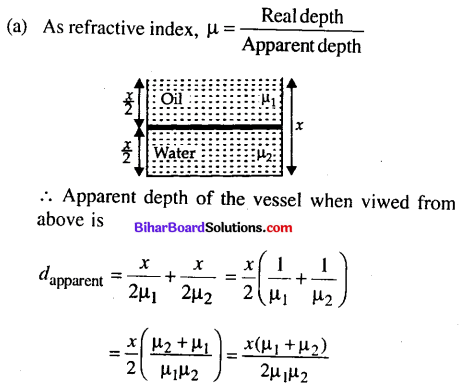Question 7.
For a total internal reflection, which of the following is corrects ?
(a) Light travel from rarer to denser medium
(b) Light travel from denser to rarer medium
(c) Light travels in air only
(d) Light travels in water only
(b) Light travel from denser to rarer medium

Question 8.
Light travels in two media A and B with speeds 1.8 x 108 m s-1 and 2.4 x 108 m s-1respectively. Then the critical angle between them is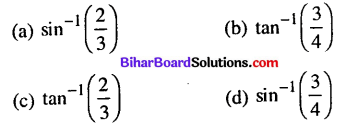(d) $$\sin ^{-1}\left(\frac{3}{4}\right)$$Question 9.
Critical angle of glass is 0j and that of water is 02. The critical angle for water and glass surface would be (μg = 3/2, μw = 4/3).
(a) less than θ2
(b) between θ1 and θ2
(c) greater than θ2
(d) less than θ1
(c) greater than θ2

Question 10.
Critical angle for light going from medium (i) to (ii) is θ. The speed of light in medium (i) is r, then the speed of light in medium (ii) is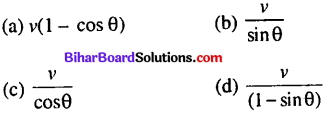(b) $$\frac{v}{\sin \theta}$$

Question 11.
Mirage is a phenomenon due to
(a) refraction of light
(b) reflection of light
(c) total internal reflection of light
(d) diffraction of light
(c) total internal reflection of light

Question 12.
A biconvex lens has a focal length 2/3 times the radius of curvature of either surface. The refractive index of the lens material is-
(a) 1.75
(b) 1.33
(c) 1.5
(d) 1.0
(a) 1.75
Solution:Question 13.
A convex lens of focal length 0.2 m and made of glass (aμg = 1.5) is immersed in water (aμw = 1.33). Find the change in the focal length of the lens.
(a) 5.8 m
(b) 0.58 cm
(c) 0.58 m
(d) 5.8 cm
(c) 0.58 m
Solution: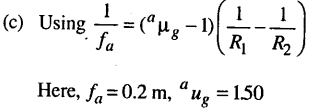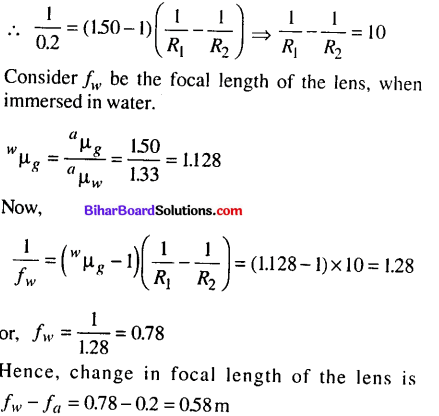Question 14.
A convex lens is dipped in a liquid whose refractive index is equal to the refractive index of the lens. Then its focal length will
(a) become zero
(b) become infinite
(c) become small, but non-zero
(d) remain unchanged
(b) become infinite

Question 15.
Radii of curvature of a converging lens are in the ratio 1 : 2. Its focal length is 6 cm and refrctive index is 1.5.
Then its radii of curvature are
(a) 9 cm and 18 cm
(b) 6 cm and 12 cm
(c) 3 cm and 6 cm
(d) 4.5 cm and 9 cm
(d) 4.5 cm and 9 cm
Solution:
(d) Here, f = 6 cm, µ = 1.5, R1 = R, R2 =- 2R According to lens maker’s formulaQuestion 16.
A converging lens is used to form an image on a screen. When the upper half of the lens is covered by an opaque screen,
(a) half the image will disappear
(b) complete image will disappear
(c) intensity of image will decrease
(d) intensity of image will increase
(c) intensity of image will decrease

Question 17.
Which of the following forms a virtual and erect image for all positions of the object ?
(a) Concave lens
(b) Concave mirror
(c) Convex mirror
(d) Both (a) and (c)
(d) Both (a) and (c)Question 18.
A real image of a distant object is formed by a plano-convex lens on its principal axis. Spherical aberration is
(a) absent.
(b) Smaller, if the curved surfaces of the lens face the object.
(c) smaller, if the plane surface of the lens faces the object.
(d) same, whichever side of the lens faces the object.
(b) Smaller, if the curved surfaces of the lens face the object.

Question 19.
A tree is 18.0 m away and 2.0 m high from a concave lens. How high is the image formed by the given lens of focal length 6m?
(a) 1.0 m
(b) 1.5 m
(c) 0.75 m
(d) 0.50 m
(d) 0.50 m
Solution:
(d) Focal length of the lens is f= -6.0 m, w = – 18 m and h = 2mQuestion 20.
The power of a biconvex lens is 10 dioptre and the radius of curvature of each surface is 10 cm. Then the refractive index of the material of the lens is
(a) $$\frac{3}{2}$$
(b) $$\frac{4}{3}$$
(c) $$\frac{9}{8}$$
(d) $$\frac{5}{3}$$
(a) $$\frac{3}{2}$$
Solution: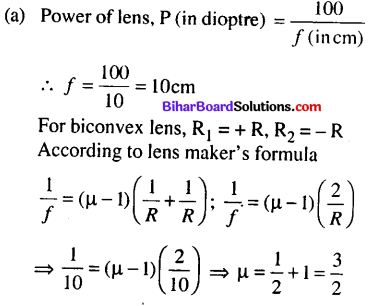Question 21.
A thin glass (refractive index 1.5) lens has optical power of-8 D in air. Its optical power in a liquid medium with refractive index 1.6 will be
(a) 1D
(b) -1D
(c) 25 D
(d) -25D
(a) 1D
Solution: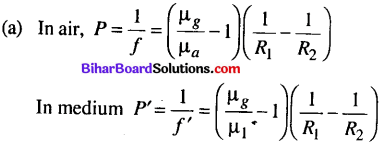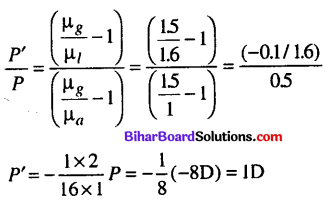Question 22.
The radius of curvature of each surface of a convex lens of refractive index 1.5 is 40 cm. It power is
(a) 2.5 D
(b) 2 D
(c) 1.5D
(d) 1 D
(a) 2.5 DQuestion 23.
Two identical glass ( μg = 3/2 ) equiconvex lenses of focal length/ are kept in contact. The space between the two lenses is filled with water μw = 4/3 ) The focal length of the combination is
(a) f
(b) $$\frac{f}{2}$$
(c) $$\frac{4 f}{3}$$
(d) $$\frac{3 f}{4}$$
(d) $$\frac{3 f}{4}$$
(d) Let R be the radius of curvature of each surface.Question 24.
A convex lens of focal length 15 cm is placed on a plane mirror. An object is placed at 30 cm from the lens. The image is
(a) real, at 30 cm in front of the mirror
(b) real, at 30 cm behind the mirror
(c) real, at 10 cm in front of the mirror
(d) virtual, at 10 cm behind the mirror
(a) real, at 30 cm in front of the mirror

Question 25.
A plano-convex lens (f = 20 cm) is silvered at plane surface. The focal length will be
(a) 20 cm
(b) 40cm
(c) 30cm
(d) 10cm
(d) 10cmQuestion 26.
A ray of light is incident at 60° on one face of a prism of angle 30° and the emergent ray makes 30° with the incident ray. The refractive index of the prism is
(a) 1.732
(b) 1.414
(c) 1.5
(d) 1.33
(a) 1.732Here, i = 60°, A = 30°, δ = 30°
As i + e = A + δ; e = A + δ – i = 30°+ 30°- 60°= 0°
Hence emergent ray in normal to the surface e = 0° ⇒ r2 = 0°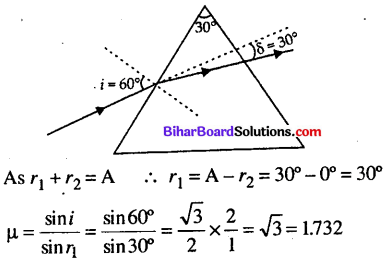Question 27.
A small angle prism (p = 1.62) gives a deviation of 4.8°. The angle of prism is
(a) 5°
(b) 6.36°
(c) 3°
(d) 7.74°
(d)7.74°
(d) Here, n= 1.62, 5 = 4.8°; μ = (μ – 1)AQuestion 28.
Which of the following colours of white light deviated most when passes through a prism ?
(a) Red light
(b) Violet light
(c) Yellow light
(d) Both (a) and (b)
(b) Violet lightQuestion 29.
White light is incident normally on a glass slab. Inside the glass slab,
(a) red light travels faster than other colours
(b) violet light travels faster than other colours
(c) yellow light travels faster than other colours
(d) all colours travel with the same speed
(a) red light travels faster than other colours

Question 30.
When light rays undergoes two internal reflection inside a raindrop, which of the rainbow is formed ?
(a) Primary rainbow
(b) Secondary rainbow
(c) Both (a) and (b)
(d) Can’t say
(b) Secondary rainbow

Question 31.
An under-water swimmer cannot see very clearly even in absolutely clear water because of
(a) absorption of light in water
(b) scattering of light in water
(c) reduction of speed of light in water
(d) change in the focal length of eye lens
(d) change in the focal length of eye lens

Question 32.
The nearer point of hypermetropic eye is 40 cm. The lens to be used for its correction should have the power
(a) + 1.5 D
(b) – 1.5 D
(c) + 2.5D
(d) + 0.5D
(c) + 2.5D
(c) Hypermetropia is corrected by using convex lens.
Focal length of lens used/= + (defected near point) f = + d = + 40 cm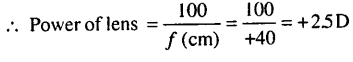Question 33.
A compound microscope consists of an objective lens with focal length 1.0 cm and eye piece of focal length 2.0 cm and a tube length 20 cm the magnification will be
(a) 100
(b) 200
(c) 250
(d) 300
(c) 250
Solution:
(c) Magnification, of compound microscopeQuestion 34.
The final image in an astronomical telescope with respect to object is
(a) virtual and erect
(b) real and erect
(c) real and inverted
(d) virtual and inverted
(d) virtual and inverted

Question 35.
An astronomical refractive telescope has an objective of focal length 20 m and an eyepiece of focal length 2 cm. Then
(a) the magnification is 1000
(b) the length of the telescope tube is 20.02 m
(c) the image formed is inverted
(d) all of these
(d) all of these

Question 36.
A giant refracting telescope at an observatory has an objective lens of focal length 15 m. If an eye piece of focal length 1.0 cm is used, what is the angular magnification of the telescope ?
(a) 1000
(b) 1500
(c) 2000
(d) 3000
(b) 1500

Question 37.
The number of capital letters such as A, B, C, D …..which are not laterally inverted by a plane mirror ?
(a) 6
(b) 7
(c) 11
(d) 13
(c) 11

Question 38.
Two mirrors at an angle 0° produce 5 images of a point. The number of images produced when 0 is decreased to 0°- 30° is
(a) 9
(b) 10
(c) 11
(d) 12
(c) 11
Solution:
(c) Here, the walls, will act as two plane mirrors inclined to each other at 90° (i.e 0 = 90°).
The number of images formed is
$$n=\frac{360^{\circ}}{90^{\circ}}-1=4-1=3$$Question 39.
A man stands symmetrically between two large plane mirrors fixed to two adjacent walls of a rectangular room. The number of images formed are
(a) 4
(b) 3
(c) 2
(d) 6
(b) 3
Solution:
(b) Different angles are as shown in figure.In triangle ABC, θ + θ + θ = 180°, θ = 60°

Question 40.
A short pulse of white light is incident from air to a glass slab at normal iiicidence. After travelling through the slab, the first colour to emerge is
(a) blue
(b) green
(c) violet
(d) red
(d) red

Question 41.
A passenger in an aeroplane shall
(a) never see a rainbow.
(b) may see a primary and a secondary rainbow as concentric cricles.
(c) may see a primary and a secondary rainbow as concentric arcs.
(d) shall never see a secondary rainbow.
(b) may see a primary and a secondary rainbow as concentric cricles.Question 42.
The phenomena involved in the reflection of radiowaves by ionosphere is similar to
(a) reflection of light by a plane mirror.
(b) total internal reflection of light in air during a mirage.
(c) dispersion of light by water molecules during the formation of a rainbow.
(d) scattering of light by the particles of air.